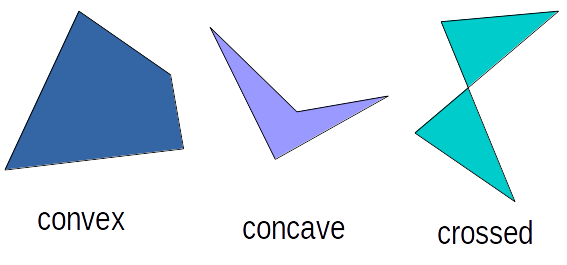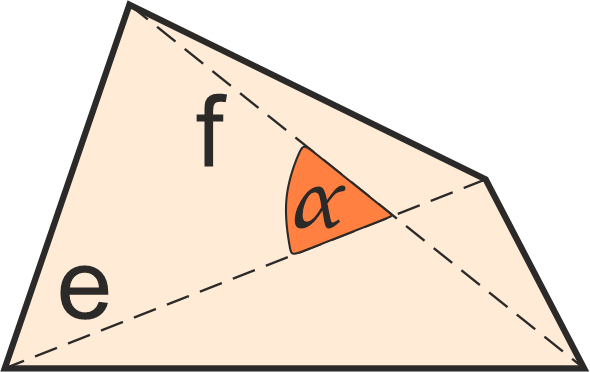Created by Hanna Pamuła, PhD candidate and Maria Kluziak
Last updated: Aug 25, 2022

If you are wondering how to find the area of any quadrilateral, check out this quadrilateral calculator. We implemented three quadrilateral area formulas, so you can find the area given diagonals and angle between them, bimedians and angle between them, or all sides and two opposite angles. In the default option you can also find the quadrilateral perimeter. If you are looking for a specific quadrilateral shape - as, for example, a rhombus or a kite - check our comprehensive list of area calculators below.

## What is a quadrilateral?

A quadrilateral is a polygon with four edges and four vertices. Sometimes it is called a quadrangle or a tetragon, by analogy to three-sided triangles and polygons with more sides (pentagon, hexagon, heptagon, octagon etc.). Quadrilaterals can be:

• simple (not self-intersecting)
1. convex - all interior angles < 180°, both diagonals lie inside the quadrilateral
2. concave - one interior angle > 180°, one diagonals lie outside the quadrilatera
• crossed, also called complex, butterflies, or bow-ties (self-intersecting)There are many types of convex quadrilaterals. The basic ones are:

• Irregular quadrilateral (UK) / trapezium (US): no sides are parallel. That's the case in which our quadrilateral area calculator is particularly useful.
• Trapezium (UK) / trapezoid (US): at least one pair of opposite sides are parallel. Isosceles trapezium (UK)/isosceles trapezoid (US) is a special case with equal base angles.
• Parallelogram: has two pairs of parallel sides.
• Rhombus or rhomb: all four sides are of equal length.
• Rectangle: all four angles are right angles.
• Square: all four sides are of equal length (equilateral), and all four angles are right angles.
• Kite: two pairs of adjacent sides are of equal length## Quadrilateral area formulas

In this calculator, you can find three ways of determining the quadrilateral area:

1. Given four sides and two opposite angles

According to Bretschneider's formula, you can calculate the quadrilateral area as:

area = √[(s - a) × (s - b) × (s - c) × (s - d) - a × b × c × d × cos²(0.5 × (angle1 + angle2))]

where a, b, c d are quadrilateral sides, s is the semiperimeter (0.5 × (a + b + c + d)), and angle1 and angle2 are two opposite angles.

1. Given diagonals and angle between them

area = p × q × sin(angle) / 2, where p, q are diagonals

1. Given bimedians and angle between them

area = m × n × sin(angle), where m, n are bimedians - lines that join the midpoints of opposite sides

1. Given the Cartesian coordinates of the vertices

If we list the vertices of our quadrilateral in the counterclockwise order:

(x1, y1), (x2, y2), (x3, y3), (x4, y4) 

then the shoelace formula tells us that the area of this quadrilateral is given by the following formula:

area = 0.5 * (x1y2 - y1x2 + x2y3 - y2x3 + x3y4 - y3x4 + x4y1 - y4x1)

## How to find the quadrilateral area?

Assume that you want to calculate the area of a parcel. It's in the quadrilateral shape, but not any specific - it's neither a rectangle, nor a trapezoid. The quadrilateral area calculator is the best choice!

1. Choose the option with your given parameters. The easiest to measure in field or on the map is the default value, with 4 sides and 2 opposite angles given. Let's pick that option.
2. Enter the given values. For example, a = 350 ft, b = 120 ft, c = 280 ft, d = 140 ft, angle1 = 70 , angle2 = 100. Remember that you can easily change the units by clicking on the unit name and selecting the one you need.
3. The quadrilateral area calculator displays the area, as well as perimeter. The area is equal to 39,259 ft2 and perimeter 890 ft in our example. Now you know how much material you need to fence the parcel.
Hanna Pamuła, PhD candidate and Maria Kluziak
unknown
Given
diagonals and angle betweenAngle α
deg
Diagonal e
in
Diagonal f
in
Area
in²
People also viewed…

### BMR - Harris-Benedict equation

Harris-Benedict calculator uses one of the three most popular BMR formulas. Knowing your BMR (basal metabolic weight) may help you make important decisions about your diet and lifestyle.

### Phase shift

The phase shift calculator is here to find the amplitude, period, phase shift, and vertical shift of an arbitrarily changed sine or cosine function.

### Steps to calories

Steps to calories calculator helps you to estimate the total amount to calories burned while walking.

### Surface area of a cylinder

How to find the surface area of a cylinder? What's the surface area of a cylinder formula? Check out this surface area of a cylinder calculator and find answers to these questions.